# 天体轨道理论¶

## 坐标¶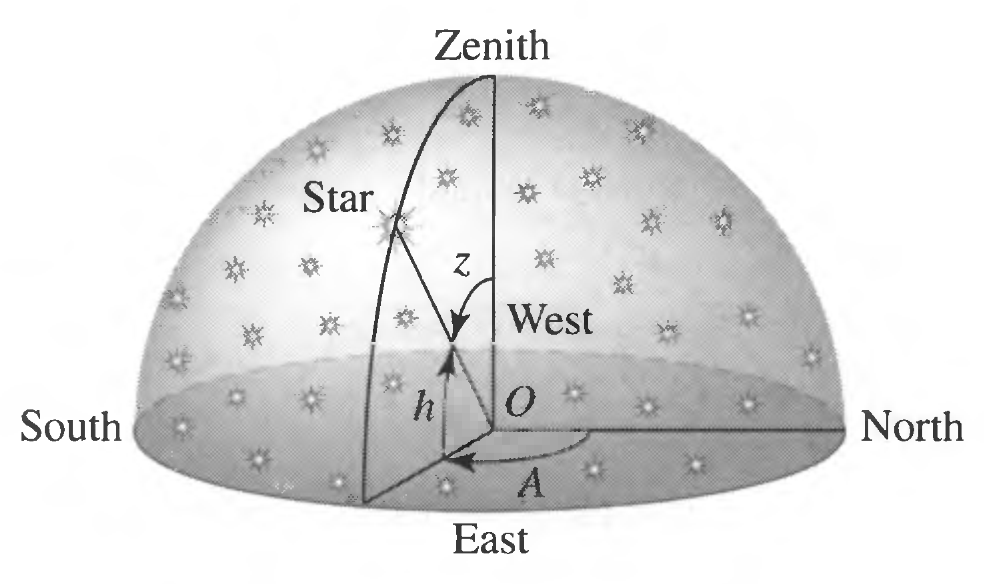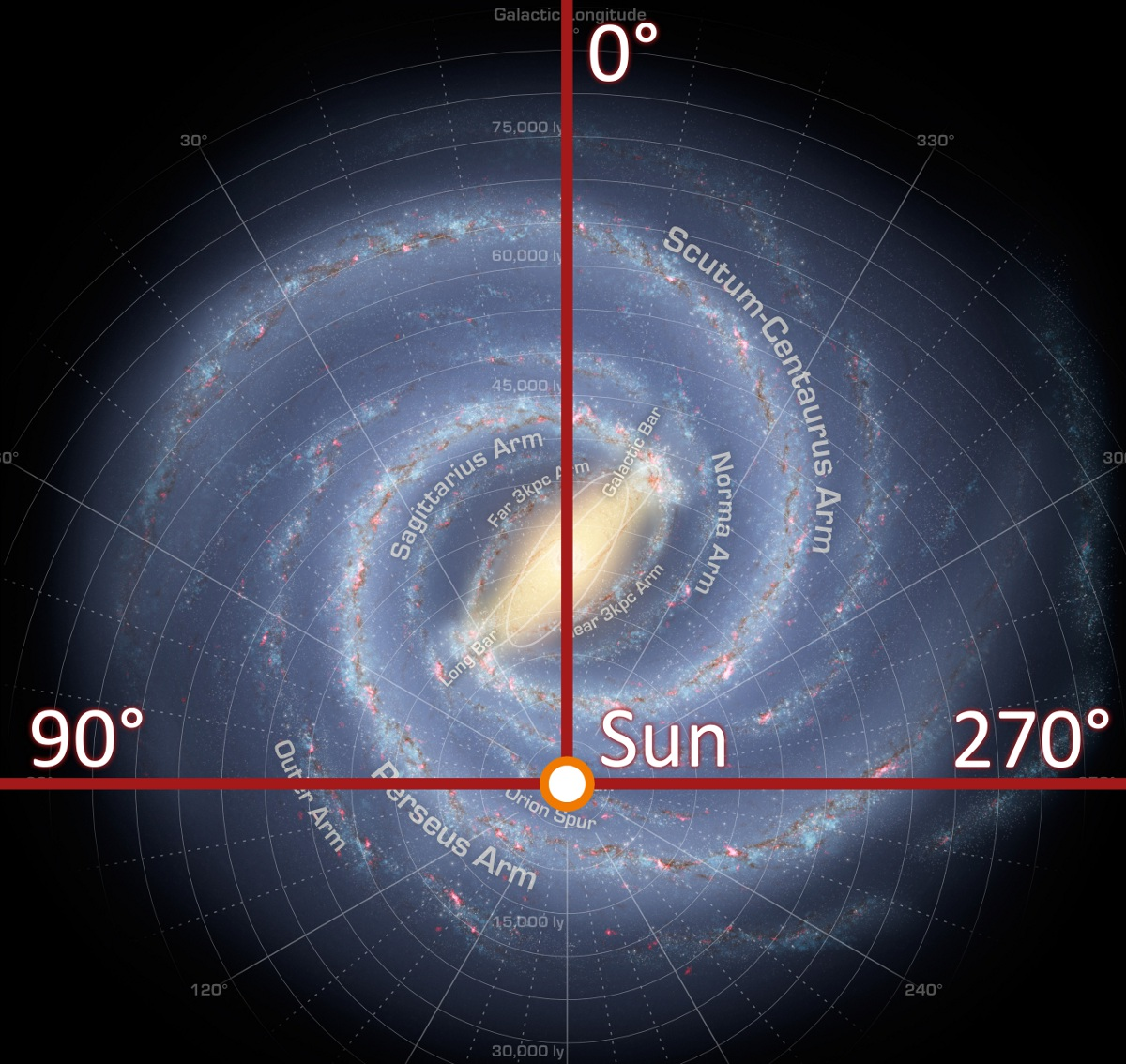## 光度和星等¶

• 光度，Luminosity，其实就是辐射功率，也就是某个面积上单位时间内通过的光的能量。例如对于恒星来说，光度就是单位时间内释放的总能量。因此单位是功率单位。通常天文上使用太阳光度作为一个参考值，$$L_{\odot} = 3.8 W$$
• 视星等，Apparent Magnitude，是一个用来表征我们在地球上观测到的天体的亮度的量。
• 绝对星等是在一个特定距离上的视星等。

$m - m_0 = -2.5 \log_{10} \left( \frac{F}{F_0} \right),$

$M = m + 5 \log_{10} \left( \frac{d_0}{d} \right)，$

M - m_0 = -2.5 log_{10} left(L/4pi d_0^2 right) + Constant，

$\begin{split}m - M &= -2.5 \log_{10} \left( L/4\pi d^2 \right) + 2.5 \log_{10} \left( L/4\pi d_0^2 \right) \\ & = -2.5 \log_{10} \left( \left(\frac{d}{d_0}\right)^2 \right) \\ & = -5 \log_{10} \frac{d_0}{d}.\end{split}$

## 开普勒定律¶

### 开普勒第一定律¶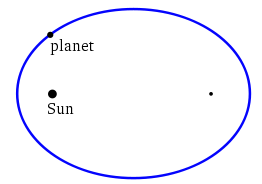### 开普勒第二定律¶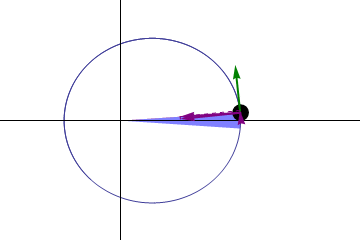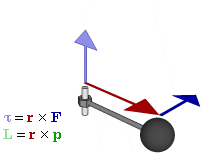实际上还跟动量和位移矢量的夹角有关，此处忽略。

### 开普勒第三定律¶

$\frac{P^2}{a^3} = \frac{4\pi^2}{GM}$

## 开普勒三定律与系外行星探测¶

1. 对于开普勒定律更加严格的描述，应该是讲“太阳”改为“质心”，同时太阳变得不那么特殊，也服从开普勒定律。因为显然我们可以取两颗质量相等的天体让他们相互围绕，这时候两颗天体没有任何一颗相对于另一颗，当我们增加其中一颗的质量然后重新让他们相互围绕的时候，在质心系看来，重的一颗的半轴会越来越小，如果我们将这颗星变成无穷大质量，那么显然质心跟这颗天体重合，所有的定律都回到了原始的开普勒定律。 对于两颗任意质量的天体的相对运动，开普勒三定律该如何修改呢？对于第一和第二定律，只需要将太阳改为质心即可。对于第三定律，我们需要替换一些变量或者重新推导。
2. 对于多星行系统，多个行星对恒星带来的影响是什么呢？
3. 在探测一个星系的时候，星系轨道平面并不是总是垂直于我们的视线方向的，那么我们如何处理这种倾斜呢？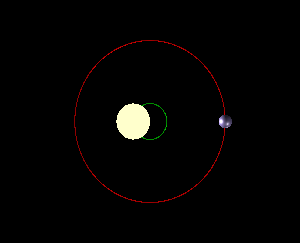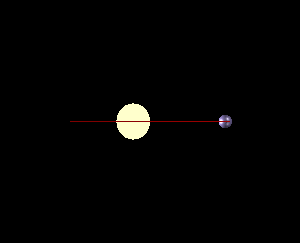### 修正开普勒三定律¶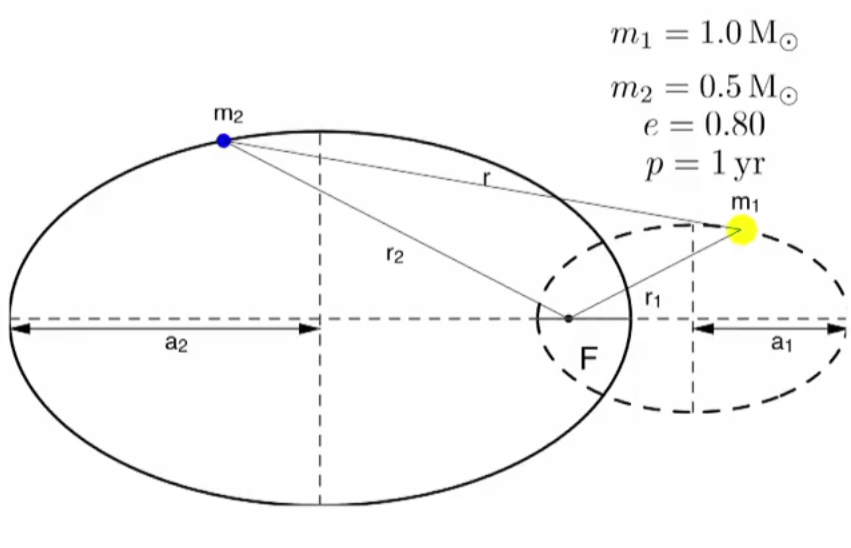Barycentric Orbit. 我们将恒星和行星整体看做一个体系，在这个体系静止参考系中，他们的质心是静止的。这样我们可以描述恒星和行星各自的轨道。

$\frac{a_1}{a_2} = \frac{m_2}{m_1}.$

$\begin{split}a_1 & = m_2 \left( \frac{P}{m_1+m_2} \right)^{2/3} \\ a_2 & = m_1 \left( \frac{P}{m_1+m_2} \right)^{2/3}\end{split}$

$m_1+m_2 = \frac{a^3_r}{P}$

$\frac{a_1}{a_2} = \frac{m_2}{m_1}.$

$\frac{a_{Solar}}{a_{Planet}} = \frac{m_{Planet}}{m_{Solar}}$

### 多星行¶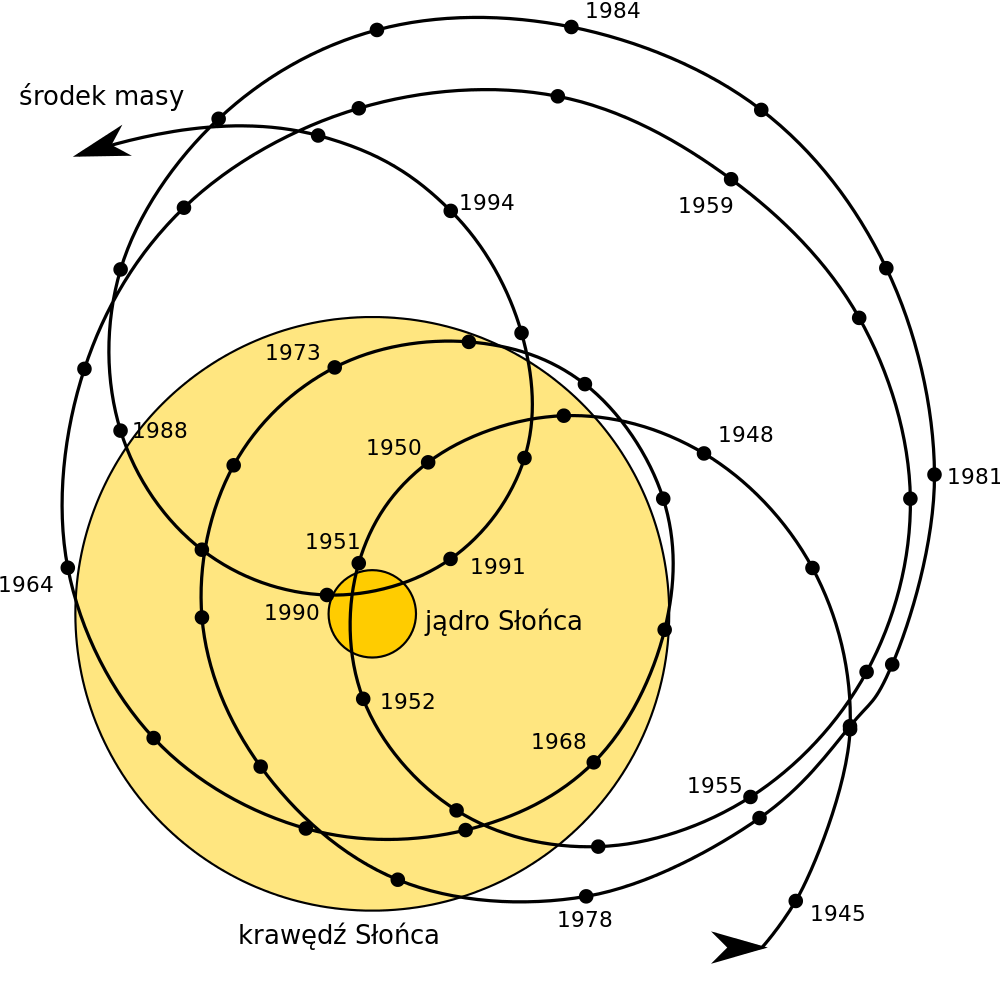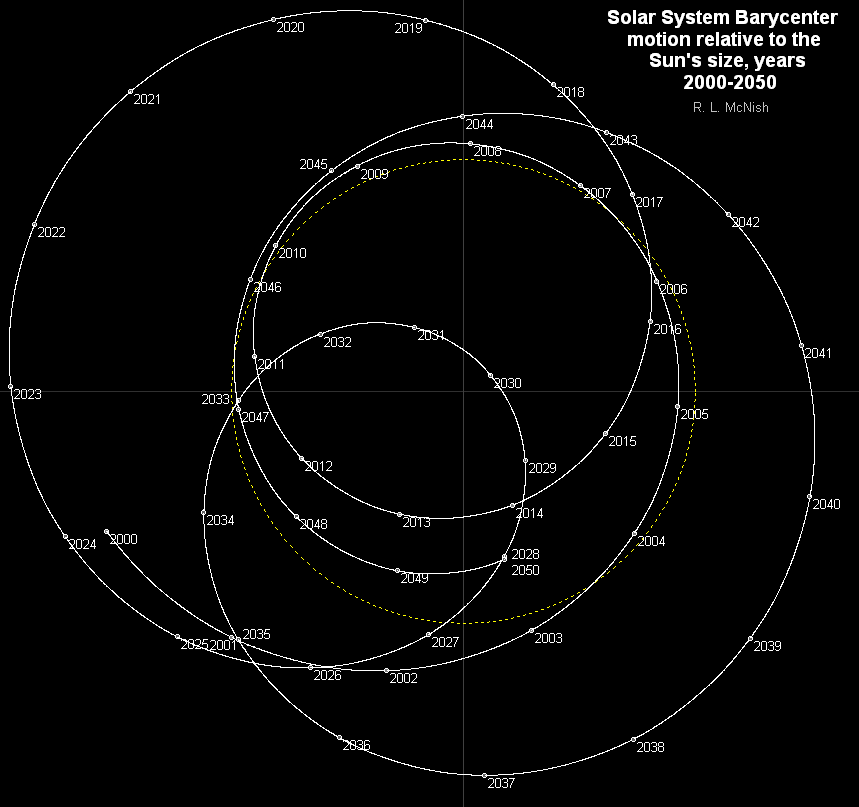### 投影平面¶## 参考和尾注¶

  开普勒定律